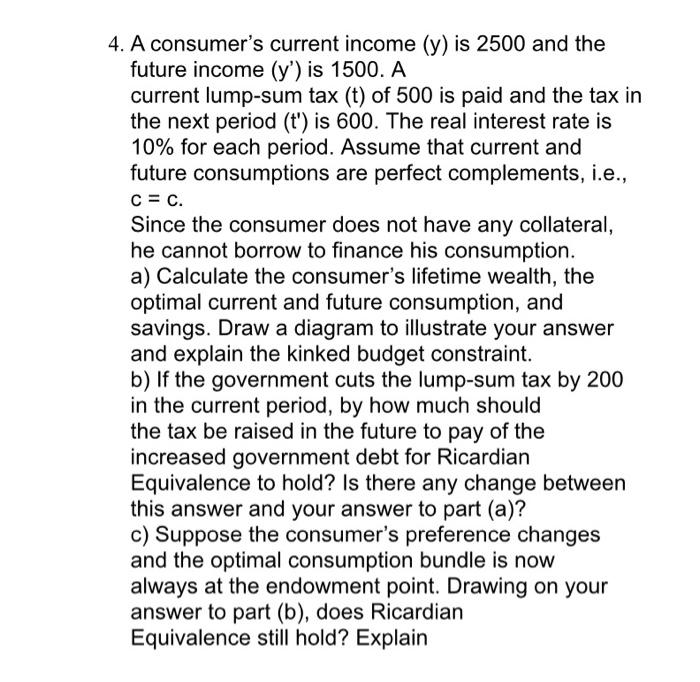Home / Expert Answers / Economics / a-consumer-39-s-current-income-mathrm-y-is-2500-and-the-future-income-left-y-prime-ri-pa901

# (Solved): A consumer's current income $$(\mathrm{y})$$ is 2500 and the future income $$\left(y^{\prime}\ri ...A consumer's current income \( (\mathrm{y})$$ is 2500 and the future income $$\left(y^{\prime}\right)$$ is $$1500 . A$$ current lump-sum tax (t) of 500 is paid and the tax in the next period ( $$\left.t^{\prime}\right)$$ is 600 . The real interest rate is $$10 \%$$ for each period. Assume that current and future consumptions are perfect complements, i.e., $$\mathrm{c}=\mathrm{c}$$. Since the consumer does not have any collateral, he cannot borrow to finance his consumption. a) Calculate the consumer's lifetime wealth, the optimal current and future consumption, and savings. Draw a diagram to illustrate your answer and explain the kinked budget constraint. b) If the government cuts the lump-sum tax by 200 in the current period, by how much should the tax be raised in the future to pay of the increased government debt for Ricardian Equivalence to hold? Is there any change between this answer and your answer to part (a)? c) Suppose the consumer's preference changes and the optimal consumption bundle is now always at the endowment point. Drawing on your answer to part (b), does Ricardian Equivalence still hold? Explain

We have an Answer from Expert